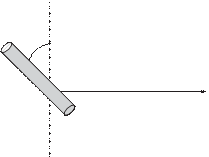### Create an Account

Already have account?

### Forgot Your Password ?

Home / Questions / A wire shown in Figure P1348 is moving in the presence of a magnetic field with B 04 Wbm2...

# A wire shown in Figure P1348 is moving in the presence of a magnetic field with B 04 Wbm2 Find the magnitude and direction of the induced voltage in the wire X X X X

A wire, shown in Figure P13.48, is moving in the presence of a magnetic field, with = 0.4 Wb/m2. Find the magnitude and direction of the inducedvoltage in the wire.

X    X    X    X     X     X     X     X     X    X    X

45°

X    X    X    X         X     X     X     X    X    X

c.    Derive the transfer function and frequency response function of the shaker mass velocity in response to the input voltage V.

Jun 18 2020 View more View Less

#### Answer (Solved)Subscribe To Get Solution